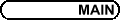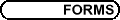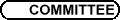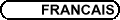Commutative Algebra / Algèbre commutative
Org: Sara Faridi (Ottawa), Sindi Sabourin (York), Will Traves (US Naval Academy) and/et Adam van Tuyl (Lakehead)

JASON BELL, University of Michigan, Department of Mathematics, 525 E. University Ave, Ann Arbor, MI 48109
Critical density in integral schemes

We say that an infinite set S of closed points of an integral scheme X is critically dense if every infinite subset of S is Zariski dense in X. This property was introduced by Rogalski, who used it to construct examples of noncommutative algebras with counter-intuitive properties.

Given a closed point p Î X and an automorphism s of X, we look at the set {sn(p) | n Î Z}. Motivated by a question of Rogalski, we explain why for sets of this form, critical density is the same as density for affine n-space over a field of characteristic 0, Fano varieties over a field of characteristic 0, and projective n-space over a field of characteristic 0. Moreover, we explain why these results do not hold in characteristic p > 0. We conclude with some open questions.

ALESSANDRA BERNARDI, Università degli studi di Milano, Via Saldini 50
Osculating varieties of Veronesean and their higher secant varieties

I want to consider the k-osculating varieties to the Veronese d-uple embeddings of Pn and study the dimension of their higher secant varieties Osk,n,d via Inverse Systems. By associating certain 0-dimensional schemes Y Ì Pn to Osk,n,d and by studying their Hilbert function it's possible, in several cases, to determine whether those secant varieties are defective or not.

RAGNAR-OLAF BUCHWEITZ, University of Toronto, Dept. of Math., 100 St. George St, Toronto, ON M5S 3G3
Free Divisors in Quiver Representations

Free divisors are reduced hypersurfaces whose singular locus is Cohen-Macaulay of codimension 2 in the ambient space. This concept was isolated first by K. Saito in the early seventies, who observed that the classical discriminants of polynomials are of this form and explained this in terms of deformation theory. In this talk, we indicate how new examples arise from the representation theory of quivers.

Parts of this talk are joint work with W. Ebeling (Hanover, Germany) and D. Mond (Warwick, UK).

MASSIMO CABOARA, Dip. di Matematica, Università di Pisa, Via Buonarroti 2, 56127 Pisa, Italy
Basic Module Operations For The Dumb

I will deal with basic module theoretic operations (syzygy computations, module intersection and colon, linear system solving) of free, finitely generated modules over k[x1,...,xn]. I will show a technique for reducing these operations to the computation of a suitable component elimination module. The algorithms resulting from this technique are easy to describe, prove and implement without any efficiency loss in comparison with the traditional algorithms.

JAYDEEP CHIPALKATTI, University of Manitoba, Winnipeg, Manitoba
Transvectants, Feynman diagrams and normality of a Brill variety

Let d=2e denote an even positive integer. Identify the space of n-ary d-ics with PN, where N = \binomd+n-1d-1. Now consider the projective subvariety

 X = {F Î PN : F = (l1  l2)e for some linear forms li }.
For historical reasons, this may be called a Brill variety. Our main result says that X is r-normal for r ³ 2 (in characteristic zero). As a consequence, one can explicitly write down defining equations for X in the case of binary forms.

The crux of the result turns out to be representation-theoretic, and is reminiscent of the Foulkes-Howe conjecture. It amounts to the assertion that a certain canonical morphism

 Symr (Symd Cn) ® Sym2 (SymreCn)
is surjective for any r ³ 2. We show this by reducing to the binary case, rephrasing it as a question involving transvectants, followed by a calculation with Feynman diagrams and hypergeometric series. This is joint work with Abdelmalek Abdesselam from Universit\' e Paris XIII.

BRIAN COOLEN, Queen's University, Kingston, Ontario, Canada
Lower Bounds and Hilbert Functions of Level Algebras

Given a polynomial ring R = k[x1,...,xn], graded by N, and a homogeneous artinian ideal I of R, then the quotient A=R/I is a graded ring that is a finite dimensional vector space over k. In particular, A can be decomposed as A = kÅA1Å¼ÅAc, where Ac ¹ 0. We let the h-vector of A be the vector (1,h1,...,hc), where hi = dimk Ai.

It is a natural question to ask which sequences of numbers (1,b1,...,bl) can be the h-vector of a graded artinian ring. This question was answered by Macaulay, who gave a test one can apply to the sequence to figure out whether it is the h-vector of a graded artinian ring or not.

However, if one imposes the condition that A must be a level algebra, then question becomes more difficult and it has not been solved yet. In general, provided one knows n, c and hc, then one can find an upper bound on the size of the hi. However, there is no corresponding lower bound.

In this talk, we shall examine this issue and compute a lower bound for the dimension of Ac-1. We shall then show that while this lower bound is not sharp, we can get very close to it. In particular, we will show that Zanello's theorem on the size of hc-1 does not extend to the case n > 7.

SUSAN COOPER, Queen's University, Kingston, Ontario K7L 3N6
Hilbert Functions Of Subsets Of Complete Intersections

A characterization of which sequences can be the Hilbert function of a finite set of distinct points in projective n-space Pn (called valid Hilbert functions for Pn) follows from the work of Macaulay, Hartshorne, and others. Although Hilbert functions of complete intersections are well-known, Hilbert functions of subsets of complete intersections have not yet been classified, even for the simplest cases. Let 1 £ d1 £ d2 £ ¼ £ dn be positive integers and H be a valid Hilbert function for Pn. We wish to determine if there exists some reduced zero-dimensional complete intersection C.I.(d1, ..., dn) which contains a subset whose Hilbert function is H.

The special case of this problem where the ideal of the complete intersection is generated by products of linear forms follows from the combinatorial work of Clements and Lindström. In general, this problem was completely answered for the case n=2 in my M.Sc. thesis and I currently have partial results for n=3. We will discuss these results as well as consider the complications that have arisen in working on this research problem. We will conclude with applications to subsets with the Cayley-Bacharach Property and extremal subsets similar to Subd(H). This work will be included in my Ph.D. dissertation.

DANIEL DAIGLE, University of Ottawa
Endomorphisms of R[X,Y] which are generically automorphic

Let R be an integral domain, K its field of fractions and X,Y indeterminates. An R-endomorphism R[X,Y] ® R[X,Y] is said to be generically automorphic if its extension K[X,Y] ® K[X,Y] is an automorphism. Let \operatornameEnd*R (R[X,Y]) be the set of R-endomorphisms of R[X,Y] which are generically automorphic. For each r Î R\{0}, define m(r) Î \operatornameEnd*R (R[X,Y]) by m(r)(X) = X and m(r)(Y) = rY.

We say that R has the weak GA-property if every element of \operatornameEnd*R (R[X,Y]) is a composition of R-automorphisms of R[X,Y] and of endomorphisms of type m(r). We partially solve the problem of identifying the class of domains having the weak GA-property. In particular, we show that an affine domain over C has the weak GA-property if and only if it is a principal ideal domain.

Joint work with Stéphane Vénéreau.

ANTHONY GERAMITA, Queen's University, Kingston, Ontario
Segre-Veronese Embeddings of P1 × P1: Secant Varieties and Fat Point Schemes in P2

If R = k[x0,x1] = Åd ³ 0 Rd is the usual graded decomposition of the polynomial ring, then the map f(d1,d2): P1 ×P1 ® Pd1 d2+d1+d2 induced by the canonical multilinear map

 Rd1 ×Rd2 ® Rd1 ÄRd2
is called the (d1,d2) Segre-Veronese embedding of P1 ×P1.

The question considered in this talk is: What are the dimensions of all the higher secant varieties to this embedding of P1 ×P1?

We show how this question is related to that of finding the Hilbert function, in degree d1 + d2, of the subscheme of P2 defined by an ideal of the form

 I = Ã1d1 ÇÃ2d2 ÇÃ32 Ç¼ÇÃs2
where: the Ãi are the ideals of points in P2; X ={P1,...,Ps} is a generic set of s points in P2; and s-2 indicates which higher secant variety we are considering.

We completely solve this problem by giving a description of the entire Hilbert function of schemes defined by ideals like I above. As a byproduct of our investigations we verify the Harbourne-Hirschowitz conjecture for this family of ideals.

This is joint work with M. V. Catalisano (Genoa) and A. Gimigliano (Bologna).

GRAHAM LEUSCHKE, Syracuse University, Syracuse, NY 13210
Endomorphism Rings of Finite Global Dimension

For a commutative local ring R, consider (possibly noncommutative) R-algebras L of the form L = EndR(M), where M is a reflexive R-module with nonzero free direct summand. Such algebras L of finite global dimension can be viewed as possible subsitutes for, or analogues of, a resolution of singularities of SpecR. For example, Van den Bergh has shown that a three-dimensional Gorenstein normal C-algebra with terminal singularities has a crepant resolution of singularities if and only if it has such an algebra L with finite global dimension and which is maximal Cohen-Macaulay over R (a "noncommutative crepant resolution of singularities"). We produce algebras L = EndR(M) having finite global dimension in two contexts: when R is a complete one-dimensional local ring, or when R is a Cohen-Macaulay ring of finite Cohen-Macaulay type. If, in the latter case, R is Gorenstein, then the construction gives a noncommutative crepant resolution of singularities in the sense of Van den Bergh.

PING LI, Queen's University, Kingston, Ontario, Canada
The Coincidence Between the Cohen-Macaulay Property and Seminormality

In the early 1970s, Hochster proved that normal semigroup rings generated by monomials are Cohen-Macaulay. When we weaken normal to seminormal, naturally, a question is raised.

Question: When is a seminormal affine semigroup ring Cohen-Macaulay? Conversely, when is a Cohen-Macaulay affine semigroup ring seminormal?

However, this question is not easily answered because for affine semigroup rings, many examples show that Cohen-Macaulay and seminormal may not coincide. For example, A=k[t2,t3] is Cohen-Macaulay but not seminormal, conversely, A=k[x,y,z2,xz,yz] is seminormal but not Cohen-Macaulay.

In this talk I will explore the coincidence between the Cohen-Macaulay property and seminormality of arbitrary affine semigroup rings, and demonstrate that under certain circumstances, an affine semigroup ring is seminormal if it is Cohen-Macaulay. Furthermore, a conjecture is given for a seminormal affine semigroup ring k[S] to be Cohen-Macaulay, and is proved when rank(S) \leqslant 3.

MOIRA McDERMOTT, Gustavus Adolphus College, 800 W. College Ave, St. Peter, MN 16082, USA
Hilbert-Kunz functions of normal local rings

This talk will report on work, joint with Craig Huneke and Paul Monsky, regarding the existence of a second coefficient in the Hilbert-Kunz function of an m-primary ideal in a normal local ring of positive characteristic. In particular, let (R, m, k) be an excellent, local, normal ring of characteristic p with a perfect residue field and dimR=d. We let n be a varying non-negative integer and let q=pn. If I is an m-primary ideal of R, and M is a finitely generated R-module, then there exists a real number b such that the length of M/I[q]M can be written as aqd +bqd-1 +O(qd-2).

CLAUDIA MILLER, Syracuse University, Department of Math, 215 Carnegie, Syracuse, NY 13244
Extremal Algebras

I will speak on joint work concerning ring homomorphisms R ® S that provide S with an R-module structure that has an extremal resolution. If S is not finitely generated, we have to generalize the definition of Betti numbers in an appropriate way. (More generally, we consider and develop the homological algebra of an S-module over the homomorphism rather than the special case N=S.) In particular, we get an example of a surprising phenomenon: that the R-module structure of S can actually give information about the rings R and S.

The ur-example of an extremal homomorphism is the Frobenius homomorphism in characteristic p > 0, but there are many others; in fact, the original motivation of this work was to show the extremality of the Frobenius.

REZA NAGHIPOUR, Department of Mathematics, University of Tabriz
Asymptotic behavior of integral closures in modules

Let R be a commutative Noetherian Nagata ring, let M be a non-zero finitely generated R-module, and let I be an ideal of R such that \operatornameheightM I > 0. In this paper there is a definition of the integral closure Na for any submodule N of M extending Rees' definition for the case of a domain. As a main result it is shown that for any submodule N of M, the sequences \operatornameAssR M/(In N)a and \operatornameAssR (In M)a / (InN)a, n = 1,2,..., of associated prime ideals, are increasing and ultimately constant for large n.

This talk will describe joint work with Peter Schenzel.

LESLIE ROBERTS, Queen's University, Kingston, Ontario K7L 3N6
Non-Cohen-Macaulay Projective Monomial Curves

We compare several ways of measuring how far a projective monomial curve is from being Cohen-Macaulay, and use these results to show that as the degree d goes to infinity the number of Cohen-Macaulay projective monomial curves of degree d grows exponentially, but the fraction of all projective monomial curves of degree d that are Cohen-Macaulay approaches 0.

This is joint work with Les Reid.

MICHAEL ROTH, Queen's University, Kingston, Ontario
A conjecture in nonmodular invariant theory.

It seems somewhat unbelievable that there would be a fact about the invariant theory of finite groups in the nonmodular case which is not well understood, but I think that there is an essential principle missing. The principle is simply the invariant theory version of Brauer's theory of relative invariants over a DVR or Dedekind domain.

The natural language to express this principle is that of algebraic geometry, and the talk will focus on explaining what the conjectured principle is, as well as some evidence for its truth, and some of its consequences.

MONIREH SEDGHI, Department of Mathematics, University of Tabriz
Asymptotic behaviour of monomial ideals on regular sequences

Let R be a commutative Noetherian ring, and let x = x1,...,xd be a regular R-sequence contained in the Jacobson radical of R. An ideal I of R is said to be a monomial ideal with respect to x if it is generated by a set of monomials x1e1¼xded. The monomial closure of I, denoted by [(I)\tilde], is defined to be the ideal generated by the set of all monomials m such that mn Î In for some n Î N. It is shown that the sequences \operatornameAssR R/[(In)\tilde] and \operatornameAssR[(In)\tilde]/In, n = 1,2,..., of associated prime ideals are increasing and ultimately constant for large n. In addition, some results about the monomial ideals and their integral closures are included.

GREGORY SMITH, Queen's University
Toric varieties as fine moduli spaces

We'll discuss how to construct projective simplicial toric varieties as the fine moduli space of representations of a quiver.

DAVID WEHLAU, Royal Military College of Canada
Separating Invariants

The concept of separating invariants, as opposed to generating invariants, was introduced a few years ago. One of the most important goals in invariant theory is to find invariants which separate group orbits. For this purpose, it is not necessary to find all invariants but only enough invariants to separate orbits. Such a set of invariants is called a set of separating invariants. Separating invariants enjoy a number of good properties and are often more well behaved then a full set of generating invariants. I will discuss some of these good properties, giving examples. I will also describe a new result (proved jointly with with H. E. A. Campbell and G. Kemper) which gives a characteristic free version of Weyl's theorem on polarization for separating invariants.